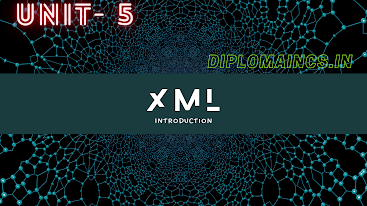## 4/14/21

### Digital Electronics and microprocessor diploam question

This question was conducted only for LE (Letral Entry) students in the month of march -2021

(Regular student's exam were postpone due to COVID-19)

Sub Code: - 1618402

Special Exam

2019(Even)

Time : 3Hrs

Semester  IV(New)/CSE

Digital Electronics and MIP

Full Marks : 70

Pass marks : 28

Group A

Choose the most suitable answer from the following options: -   (1*20=20)

(i) The decimal number 17 is equal to the binary number

(a) 10010

(b) 11000

(c)  10001

(d) 01001

(ii) The main advantage of ECL over TTL or CMOS is

(a) ECL is less expensive

(b) ECL consume less power

(c) ECL is faster

(d) None of these

(iii) The output of a gate is LOW when at least of inputs is HIGH. This is true for

(a) AND

(b) NAND

(c) OR

(d) NOR

(iv) According to DeMorgan’s theorems the following equality (s) are correct.

(a)(b)(c)(d) All of these

(v) A flip-flop is in toggle condition when

(a) J=1, K=0

(b) J=1,K=1

(c) J=0,K=0,

(d)J=0,K=1

(vi) A stage in a shift register consists of:

(a) A latch

(b) A flip-flop

(c) A byte of storage

(d) Four bits of storage

(vii) The NAND or NOR gates are referred to as “universal gate” because either

(a) Cab be found almost all digital circuits

(b) Can be used to build all the other types of gates

(c) Are used in all countries of the world

(d)Were the first gates to be integrated

(viii) An synchronous counter differs from a synchronous counter in

(a) The number of steps in its sequence

(b) The method of clocking

(c) The type of flip-flop used

(d) The value of the modulus

(ix) How many flip-flop are required to construct a decode counter?

(a) 10

(b) 8

(c) 5

(d) 4

(x) If A and B are the two inputs to the half adder then the expression for sum is;

(a) A XOR B

(b) A XNOR B

(c) A AND B

(d)A OR B

(xi) One of the methods is used for digital to analog conversion is:

(b) Successive approximation method

(c) Parallel comparator

(d) None of these

(xii) A flash memory

(a) Volatile

(b) Non-volatile

(d)  (b) and (c)

(xiii) A RAM is a

(a) Non-volatile memory

(b) Volatile memory

(d) b and c

(xiv) In general a de-multiplexer has

(a)One data input, several data output and selection inputs

(b) Several data inputs, several data outputs and one selection outputs

(c) Several data inputs, several data outputs and selection outputs

(d) Several data inputs, one data outputs and selection outputs

(xv) The device used to convert binary number to a 7 segment display format is-

(a)  Multiplexer

(b) Encoder

(c)  Decoder

(d) Register

(xvi) Data selector are basically the same as

(a)  Decoders

(b) De-multiplexers

(c)  Multiplexers

(d) Encoders

(xvii) The software used to drive microprocessor based system is called

(a)  Assembly language

(b) Firmware

(c)  Machine language code

(d) Basic interpreter instructions

(xviii) Which bus is a bidirectional bus?

(b) Data bus

(c)  Both a and b

(d) None of these

(xix) The 8085 instruction JMP comes in-

(a)  Machine control group

(b) Branch group

(c)  Arithmetic group

(d) Data transfer group

(xx) The device that provides the means for a computer to communicate with the user or other computers are referred as:

(a)  CPU

(b) ALU

(c)  Input/output

(d) None of these

Group:-"B"

Answer all Five Questions: -                         (5*4=20)

2.  Simply the following expression:

1.2.OR

Write down the addressing mode of 16 bit 8086 microprocessor.

3. With the help of truth table, explain octal binary encoder.

OR

State and explain DeMorgan’s theorem.

4. Represent the decimal number 27 in binary form using-

2.    Gray code

OR

Explain D-flip flop with its truth table.

5.  Explain the operation of ROM(Read Only Memory)

OR

Explain the following Instruction set of 8085 microprocessor

a.          XRA

b.          DCR

6. Differentiate Asynchronous and synchronous counter with examples.

OR

Define figure or merit and Fan-out of digital ICs.

Group:- "C"

Answer all Five Questions: -                         (5*6=30)

7.  Minimize the following using k-map, where d is don’t case

F(A,B,C,D)=Σ m(1,3,7,11,15) + (0,2,5)

OR

Explain JK flip-flop with its truth table. When the race round condition in JK flip flop occurs.

8.  Draw a block diagram of 4:1 multiplexer. Explain it with truth table and also write expression for output.

OR

Define opcode and operand and specify the operand in the instruction

MOV H, L

9.  Represent (-17)10 in the following representation

1.        Sign magnitude

2.       One’s complement

3.       Two’s complement

OR

Draw the logic diagram, truth table for a three flip-flop ripple counter.

10.  With the help of truth table, explain half adder and realize sum and carry using NAND gate.

OR

Explain Jump instruction. Write down various condition Jump instruction.

11.  Write symbol and truth table for the following two inputs gates.

a.          XOR gate

b.          NAND gate

c.          XNOR gate

OR

Write short notes on any two

a.    Shift register

b.    Random Access Memory(RAM)

c.     Analog digital converter

d.    Excess-3 code